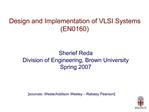# Design and Implementation of VLSI Systems EN0160 - PowerPoint PPT PresentationDownload PresentationDesign and Implementation of VLSI Systems EN0160

Design and Implementation of VLSI Systems EN0160
Download Presentation## Design and Implementation of VLSI Systems EN0160

- - - - - - - - - - - - - - - - - - - - - - - - - - - E N D - - - - - - - - - - - - - - - - - - - - - - - - - - -
##### Presentation Transcript

2. Lecture05: MOS transistor theory Last time Gate layouts and stick diagrams This time MOS transistor theory (ideal case)

3. gate-oxide-body sandwich = capacitor

4. The MOS transistor has three regions of operation Cut off Vgs < Vt

5. How to calculate the current value? MOS structure looks like parallel plate capacitor while operating in inversion Gate � oxide � channel Qchannel = CV C = eoxWL/tox = CoxWL (where Cox=eox/tox) V = Vgc � Vt = (Vgs � Vds/2) � Vt

6. Carrier velocity is a factor in determining the current Charge is carried by electrons Carrier velocity v proportional to lateral E-field between source and drain v = �E � called mobility E = Vds/L Time for carrier to cross channel: t = L / v

7. I=Q/t Now we know How much charge Qchannel is in the channel How much time t each carrier takes to cross

8. In linear mode (Vgs > Vt & Vds < Vgs-Vt)

9. In saturation mode (Vgs > Vt and Vds = Vgs-Vt)

10. Operation modes summary

11. PMOS is similar

12. What happens when we construct a INV (PMOS+NMOS)?

13. Inverter voltage transfer function

14. Summary This lecture Ideal transistor modeling Next lecture Non ideal transistor modeling

15. Inverter current transfer function